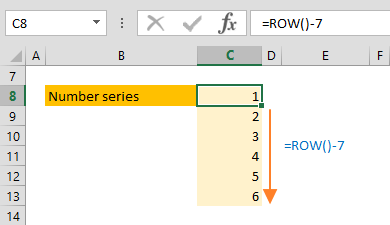The Excel ROW function is a Lookup & Reference formula that returns the row number of a reference. In this guide, we’re going to show you how to use the Excel ROW function and also go over some tips and error handling methods.

# Supported versions

• All Excel versions

ROW([reference])

# Arguments

 [reference] Optional. A valid reference.

Examples

## Simple Scenario

The ROW function without an argument returns the number of the row containing the formula.

=ROW()

If a valid cell reference is entered as an argument, the return value will be the number of the reference row.

=ROW(C2)

If you enter a range reference, you will get the number of the top row. When the dynamic arrays are released, you will get the numbers of all rows in the range reference.

=ROW(C2:E4)## Generating number series

To find the row number of a reference, you can use the ROW function in multiple adjacent rows, and get a series of numbers. In our example, we placed the ROW function in row 8, and subtracted 7 from the row number. This gives us number 1 for the row 8. If we copy same formula to the row 13, we get a series of numbers from 1 to 6.# Tips

• Use the ROWS function if you need to count the number of rows in a reference.
• To get the column number of a reference, use the COLUMN function.